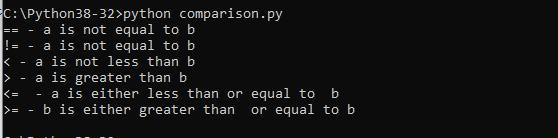Home » Comparison operators in Python

# Comparison operators in Python

Comparison operators are used to compare two values

 Operator Description == If the values of the two operands are equal, then the condition is true. != If the values of the two operands are not equal, then condition is true. > If the value of the left operand is greater than the value of the right operand, then condition is true. < If the value of the left operand is less than the value of the right operand, then the condition is true. >= If the value of the left operand is greater than or equal to the value of the right operand, then condition is true. <= If the value of the left operand is less than or equal to the value of the right operand, then the condition is true.

Lets look at an example of the comparison operators

```#!/usr/bin/python
​
a = 50
b = 40
c = 0
​
if ( a == b ):
print ("== - a is equal to b")
else:
print ("== - a is not equal to b")
​
if ( a != b ):
print ("!= - a is not equal to b")
else:
print ("!= - a is equal to b")
​
if ( a < b ):
print ("< - a is less than b" )
else:
print ("< - a is not less than b")
​
if ( a > b ):
print ("> - a is greater than b")
else:
print ("> - a is not greater than b")
​
a = 40;
b = 50;
if ( a <= b ):
print ("<= - a is either less than or equal to b")
else:
print ("<= - a is neither less than nor equal to b")
​
if ( b >= a ):
print (">= - b is either greater than or equal to b")
else:
print (">= - b is neither greater than nor equal to b")```

This is the output you should see running this example0 comment

#### You may also like

This website uses cookies to improve your experience. We'll assume you're ok with this, but you can opt-out if you wish. Accept Read More